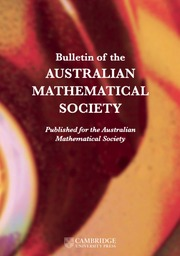Home
Hostname: page-component-7f7b94f6bd-q7wkk Total loading time: 0.364 Render date: 2022-06-30T15:07:54.645Z Has data issue: true Feature Flags: { "shouldUseShareProductTool": true, "shouldUseHypothesis": true, "isUnsiloEnabled": true, "useRatesEcommerce": false, "useNewApi": true } hasContentIssue trueBulletin of the Australian Mathematical Society

# A NEW$\boldsymbol {q}$-ANALOGUE OF VAN HAMME’S (A.2) SUPERCONGRUENCE

Published online by Cambridge University Press:  20 May 2022

## Abstract

We give a new q-analogue of the (A.2) supercongruence of Van Hamme. Our proof employs Andrews’ multiseries generalisation of Watson’s$_{8}\phi _{7}$ transformation, Andrews’ terminating q-analogue of Watson’s$_{3}F_{2}$ summation, a q-Watson-type summation due to Wei–Gong–Li and the creative microscoping method, developed by the author and Zudilin [‘A q-microscope for supercongruences’, Adv. Math.346 (2019), 329–358]. As a conclusion, we confirm a weaker form of Conjecture 4.5 by the author [‘Some generalizations of a supercongruence of van Hamme’, Integral Transforms Spec. Funct.28 (2017), 888–899].

## MSC classification

Type
Research Article
© The Author(s), 2022. Published by Cambridge University Press on behalf of Australian Mathematical Publishing Association Inc.

## Access options

Get access to the full version of this content by using one of the access options below. (Log in options will check for institutional or personal access. Content may require purchase if you do not have access.)

## References

Andrews, G. E., ‘Problems and prospects for basic hypergeometric functions’, in Theory and Application for Basic Hypergeometric Functions, Mathematics Research Center, the University of Wisconsin–Madison, 35 (ed. Askey, R. A.) (Academic Press, New York, 1975), 191224.Google Scholar
Andrews, G. E., ‘On$q$-analogues of the Watson and Whipple summations’, SIAM J. Math. Anal. 7 (1976), 332336.CrossRefGoogle Scholar
Gasper, G. and Rahman, M., Basic Hypergeometric Series, 2nd edn, Encyclopedia of Mathematics and Its Applications, 96 (Cambridge University Press, Cambridge, 2004).CrossRefGoogle Scholar
Guo, V. J. W., ‘Some generalizations of a supercongruence of Van Hamme’, Integral Transforms Spec. Funct. 28 (2017), 888899.CrossRefGoogle Scholar
Guo, V. J. W., ‘A$q$-analogue of the (A.2) supercongruence of Van Hamme for primes${p\equiv 1 \pmod 4}$’, Rev. R. Acad. Cienc. Exactas Fís. Nat. Ser. A Mat. RACSAM 114 (2020), Article no. 123.Google Scholar
Guo, V. J. W. and Schlosser, M. J., ‘Some$q$-supercongruences from transformation formulas for basic hypergeometric series’, Constr. Approx. 53 (2021), 155200.CrossRefGoogle Scholar
Guo, V. J. W. and Zudilin, W., ‘A$q$-microscope for supercongruences’, Adv. Math. 346 (2019), 329358.CrossRefGoogle Scholar
Guo, V. J. W. and Zudilin, W., ‘A common$q$-analogue of two supercongruences’, Results Math. 75 (2020), Article no. 46.CrossRefGoogle Scholar
Guo, V. J. W. and Zudilin, W., ‘A q-microscope for supercongruences’, Adv. Math. 346 (2019), 329358.CrossRefGoogle Scholar
Liu, J.-C., ‘On Van Hamme’s (A.2) and (H.2) supercongruences’, J. Math. Anal. Appl. 471 (2019), 613622.CrossRefGoogle Scholar
McCarthy, D. and Osburn, R., ‘A$p$-adic analogue of a formula of Ramanujan’, Arch. Math. (Basel) 91 (2008), 492504.CrossRefGoogle Scholar
Robert, A. M., A Course in$p$-Adic Analysis, Graduate Texts in Mathematics, 198 (Springer-Verlag, New York, 2000).CrossRefGoogle Scholar
Swisher, H., ‘On the supercongruence conjectures of Van Hamme’, Res. Math. Sci. 2 (2015), Article no. 18.CrossRefGoogle Scholar
Van Hamme, L., ‘Proof of a conjecture of Beukers on Apéry numbers’, in Proceedings of the Conference on$p$-Adic Analysis, Houthalen, 1987 (eds. N. De Grande-De Kimpe and L. Van Hamme) (Vrije Universiteit Brussel, Brussels, 1986), 189195.Google Scholar
Van Hamme, L., ‘Some conjectures concerning partial sums of generalized hypergeometric series’, in$p$-Adic Functional Analysis, Nijmegen, 1996, Lecture Notes in Pure and Applied Mathematics, 192 (eds. W. H. Schikhof, C. Perez-Garcia and J. Kakol) (Dekker, New York, 1997), 223236.Google Scholar
Wang, X. and Yue, M., ‘A$q$-analogue of the (A.2) supercongruence of Van Hamme for any prime$p\equiv 3 \pmod 4$’, Int. J. Number Theory 16 (2020), 13251335.CrossRefGoogle Scholar
Wei, C., ‘Some$q$-supercongruences modulo the fourth power of a cyclotomic polynomia’, J. Combin. Theory Ser. A 182 (2021), Article no. 105469.Google Scholar
Wei, C., ‘Some$q$-supercongruences modulo the fifth and sixth powers of a cyclotomic polynomial’, Preprint, 2021, arXiv:2104.07025.CrossRefGoogle Scholar
Wei, C., Gong, D. and Li, J., ‘Summation formulae for$q$-Watson type${}_4{\phi}_3$-series’, Discrete Math. 313 (2013), 15891593.CrossRefGoogle Scholar

# Save article to Kindle

Note you can select to save to either the @free.kindle.com or @kindle.com variations. ‘@free.kindle.com’ emails are free but can only be saved to your device when it is connected to wi-fi. ‘@kindle.com’ emails can be delivered even when you are not connected to wi-fi, but note that service fees apply.

Find out more about the Kindle Personal Document Service.

A NEW$\boldsymbol {q}$-ANALOGUE OF VAN HAMME’S (A.2) SUPERCONGRUENCE Available formats × # Save article to Dropbox To save this article to your Dropbox account, please select one or more formats and confirm that you agree to abide by our usage policies. If this is the first time you used this feature, you will be asked to authorise Cambridge Core to connect with your Dropbox account. Find out more about saving content to Dropbox. A NEW$\boldsymbol {q}$-ANALOGUE OF VAN HAMME’S (A.2) SUPERCONGRUENCE
Available formats
×

# Save article to Google Drive

To save this article to your Google Drive account, please select one or more formats and confirm that you agree to abide by our usage policies. If this is the first time you used this feature, you will be asked to authorise Cambridge Core to connect with your Google Drive account. Find out more about saving content to Google Drive.

A NEW$\boldsymbol {q}\$-ANALOGUE OF VAN HAMME’S (A.2) SUPERCONGRUENCE
Available formats
×
×